# Stokes Polarization Parameters

### Limitations of Polarization Ellipse

As introduced in the article of "polarization states" and Jones Matrix (click to learn more), polarization ellipse is very useful since we can study the various states of polarized light from a single equation.

Linearly polarized light and circularly polarized light are shown to be just special cases of polarization ellipse.

But, there two serious limitations of polarization ellipse (also Jones matrix calculus):

1. Lights are of very high frequencies, a single period of vibration is in a time interval of the order 10-15 sec. We cannot actually observe the polarization ellipse.
2. The polarization ellipse can only describe completely polarized light. It can not be used to describe partially polarized nor unpolarized light. But in practice, most lights are partially polarized.

Polarization ellipse is an idealization of the true behavior of light; it is only correct at any given instant of time which we cannot observe directly.

Thus, in practice we have to describe light with observed or measured quantities. We can only use time averaged values of the optical field that we can observe.

in 1852, Sir George Gabriel Stokes (1819 - 1903, mathematician, physicist, politician and theologian of Ireland), found that any state of polarized light could be completely described by four measurable quantities:

1. The total intensity of the light (polarized + unpolarized) S0
2. The intensity of linear horizontal or vertical polarization S1
3. The intensity of linear +45° or -45° polarization S2
4. The intensity of right or left circular polarization S3

The intensity of the polarized part of the lightwave is given by:The total intensity S0 isThus: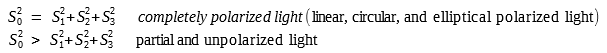### The Derivation of Stokes Polarization Parameters

We consider two plane waves that are orthogonal to each other at a point in space (x, y, z). Without losing generality,  we can set z = 0.

These two waves which may not necessarily be monochromatic can be written in equations:where E0x(t), E0y(t) are the instantaneous amplitudes, ω is the instantaneous angular frequency, δx(t), δy(t) are the instantaneous phase factors.

Comparing to the super fast vibrations of the waves (cosinusoids), the amplitudes  E0x(t), E0y(t) and phase factors δx(t), δy(t) fluctuate very slowly. Here, we are considering monochromatic waves, and their amplitudes and phase factors are constant, not changing with time. So we can use them as E0x, E0y, δx, δy.

By removing the term ωt in (1) and (2), we then get the familiar polarization ellipse:where δ = δy - δx

In order to represent (3) in terms of the observables of the optical field, we must take an average over the time of observation.

Since the vibration is so fast, the observation time can be seen as infinite. But, since Ex(t) and Ey(t) are both periodical, we can actually average (3) only over a single oscillation period.

The time average is represented by the angular brackets <...>, so (3) can written as: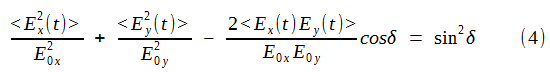where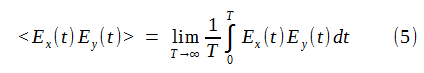We then multiple (4) by 4E0x2E0y2, and then get: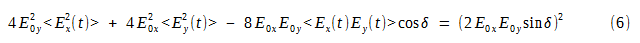From (1) and (2), we can find the average values of Equation (6) using Equation (5)Substituting (7), (8), (9) into (6) and we get:Since we want to express the final result in terms of intensity, we can add and subtract the quantity E0x4 + E0y4 to the left-hand side of (10); doing this yields perfect squares.

Then we can get: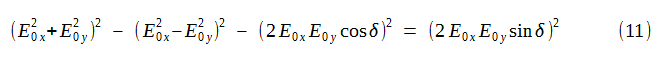We write the quantities inside the parentheses as:Note that S0, S1, S2, S3 are time-averaged quantities performed over a time interval τD that is the characteristic time constant of the detection process.

We then rewrite (11) as: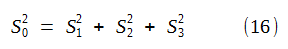The four equations (12), (13), (14) and (15) are the Stokes polarization parameters for a plane wave.

Note:

1. Stokes parameters are expressed in terms of intensities (which we can measure)
2. Stokes parameters are real quantities (instead of complex numbers as in Jones matrices)

If we have partially polarized light, equation (12) is still true for very short time intervals since the amplitudes and phases fluctuate slowly.

Based on Schwartz's inequality, we can say that for any state of polarized light:In (17), the equality is true for completely polarized light, and inequality is true for partially polarized or unpolarized light.

### Stokes parameters and polarization ellipse

From this article about polarization states and polarization ellipse, we can see the orientation angle ψ of the polarization ellipse is given by: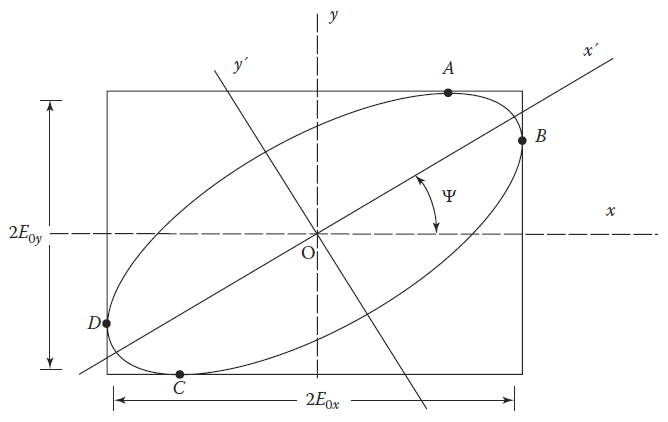When we take a close look at (12), (13), (14) and (15), we find out that if we divide (14) by (13), ψ can be written as expressions of Stokes parameters as:Also from the same article, we see that another important parameter is the ellipticity angle χ of the polarization ellipse, which is given by:After some calculation, the final result of χ is expressed with amplitudes and phase factor as: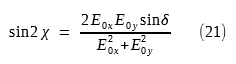We found out that we can divide (15) by (12) and rewrite χ as an expression of Stokes parameters: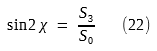### Degree of Polarization (DOP) in terms of Stokes Parameters

We can use Stokes parameters to describe the degree of polarization for any state of polarization (completely polarized, partially polarized, and unpolarized).

The Degree of Polarization P is defined as (based on light intensity):where Ipol is the intensity of the sum of the polarization components, and Itot is the total intensity of the beam.

So the meaning of P is:

• P = 1          ---> completely polarized light
• P = 0          ---> unpolarized light
• 0 < P < 1    ---> partially polarized light

### Complex Amplitudes representation of Stokes Parameters

We usually use complex amplitudes to represent the real optical amplitudes. So equation (1) and (2) can be written as: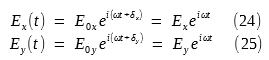where complex amplitudes Ex, Ey are defined as:We now can get the Stokes parameters for a plane wave from the formulas below: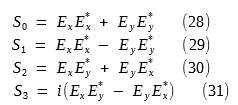where Ex* and Ey* are the complex conjugates of Ex and Ey respectively.

From now on, we will use the above definitions expressed in complex amplitudes for the Stokes parameters.

### Stokes Parameters Examples for Completely Polarized Light

We now discuss Stokes parameters for some special cases.

#### 1. Linear Horizontally Polarized Light (LHP)

For this case, there is no vertical field component, so E0y = 0. From (12), (13), (14) and (15) we get: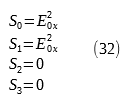#### 2. Linear Vertically Polarized Light (LVP)

For this case, there is no horizontal field component,  so E0x = 0. From (12) to (15) we get: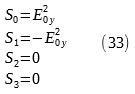#### 3. Linear +45° Polarized Light (L +45)

The condition for obtaining linear +45° polarized light are:

• E0x = E0y = E0
• δ = δy - δx = 0

This means this polarization is a superposition of in-phase, equal-amplitude horizontal and vertical fields. With (12) to (15), we get: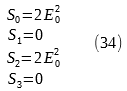#### 4. Linear -45° Polarized Light (L -45)

The condition for obtaining linear -45° polarized light are:

• E0x = E0y = E0
• δ = δy - δx = 180°

We then get: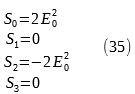#### 5. Right Circularly Polarized Light (RCP)

The condition for obtaining right circularly polarized light are:

• E0x = E0y = E0
• δ = δy - δx = 90°

We then get: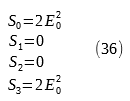#### 6. Left Circularly Polarized Light (LCP)

The condition for obtaining left circularly polarized light are:

• E0x = E0y = E0
• δ = δy - δx = - 90°

We then get: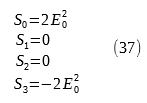#### 7. Elliptically Polarized Light

The Stokes parameters for general elliptically polarized light are as the definition:### Stokes Parameters for Unpolarized Light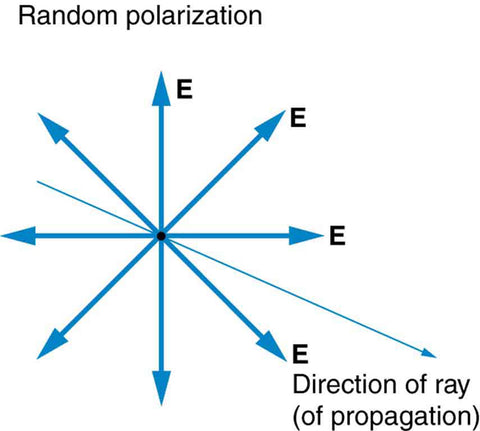In order to understand Stokes parameters for unpolarized and partially polarized light, please read this tutorial on how to measure Stokes parameters.

From the article about how to measure Stokes parameters, we saw that the intensity I(φ,θ) of a light beam emerging from the retarder/polarizer pair was: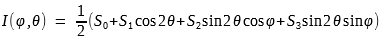Unpolarized light has no preference over any particularly linear polarization direction as shown in the previous picture.

The intensity I(φ,θ) is unaffected by either the presence of a retarder (φ) or rotation of the linear polarizer (θ), and there is no polarized part in the intensity. Thus we have: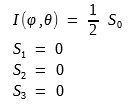And the Stokes vector for unpolarized light is: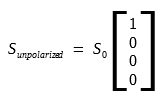### Stokes Parameters for Partially Polarized Light

Partially polarized light is a mixture of completely polarized light and unpolarized light.

According to (23), the Degree of Polarization P is defined as:The Stokes vector for partially polarized light is: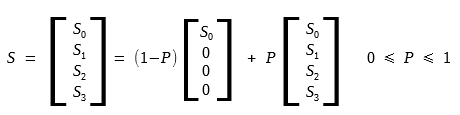For completely polarized light, P = 1, and the above equation reduces to the Stokes vector for elliptically polarized light.

Similarly, for unpolarized light, P = 0, and the above equation reduces to the Stokes vector for unpolarized light.